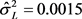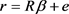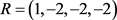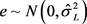﻿ 基于先验信息的改进随机约束两参数估计

# 基于先验信息的改进随机约束两参数估计Improved Stochastic Restricted Two-Parameter Estimation Based on Prior Information

Abstract: This paper mainly aims at the situation where there is complex collinearity in the general linear model. Combining the mixed estimator under the condition of stochastic restricted and the two-parameter estimator of parameter vectors based on prior information, a new biased estimator is proposed, that is, the stochastic constrained modified two-parameter estimator. In the sense of mean square error matrix criterion, the superiority of stochastic constrained modified two-parameter estimator relative to two-parameter estimator, modified two-parameter estimator, mixed estimator, stochastic restricted ridge estimator and stochastic restricted two-parameter estimator are studied. Further, the selection of bias parameters is discussed. At the same time, the performance of the proposed estimator is explained through a numerical example.

1. 介绍

$y=X\beta +\epsilon$ (1)

${\stackrel{^}{\beta }}_{L}={\left({X}^{\prime }X\right)}^{-1}{X}^{\prime }y$ (2)

$\stackrel{^}{\beta }\left(k\right)={\left({X}^{\prime }X+kI\right)}^{-1}{X}^{\prime }X{\stackrel{^}{\beta }}_{L}$ (3)

$\stackrel{^}{\beta }\left(d\right)={\left({X}^{\prime }X+I\right)}^{-1}\left({X}^{\prime }X+dI\right){\stackrel{^}{\beta }}_{L}$ (4)

$\stackrel{^}{\beta }\left(k,d\right)={\left({X}^{\prime }X+kI\right)}^{-1}\left({X}^{\prime }X+kd\right){\stackrel{^}{\beta }}_{L}$ (5)

$\stackrel{^}{\beta }\left(k,b\right)={\left({X}^{\prime }X+kI\right)}^{-1}\left({X}^{\prime }X{\stackrel{^}{\beta }}_{L}+kb\right)$ (6)

$\stackrel{^}{\beta }\left(d,b\right)={\left({X}^{\prime }X+I\right)}^{-1}\left(\left({X}^{\prime }X+dI\right){\stackrel{^}{\beta }}_{L}+\left(1-d\right)b\right)$ (7)

$\stackrel{^}{\beta }\left(k,d,b\right)={\left({X}^{\prime }X+kI\right)}^{-1}\left(\left({X}^{\prime }X+kd\right){\stackrel{^}{\beta }}_{L}+k\left(1-d\right)b\right)$ (8)

$r=R\beta +e$ (9)

Theil和Goldberger  通过构建下面的增广矩阵模型：

$\left(\begin{array}{c}y\\ r\end{array}\right)=\left(\begin{array}{c}X\\ R\end{array}\right)\beta +\left(\begin{array}{c}\epsilon \\ e\end{array}\right)$ (10)

${y}_{m}={X}_{m}\beta +{u}_{m}$ (11)

${\stackrel{^}{\beta }}_{m}={\left(S+{R}^{\prime }{V}^{-1}R\right)}^{-1}\left({X}^{\prime }y+{R}^{\prime }{V}^{-1}r\right)$ (12)

${\Psi }_{1}={\left({y}_{m}-{X}_{m}\beta \right)}^{\prime }\left({y}_{m}-{X}_{m}\beta \right)+{\left(r-R\beta \right)}^{\prime }{V}^{-1}\left(r-R\beta \right)$

${\stackrel{^}{\beta }}_{SSRE}={\left(S+{R}^{\prime }{V}^{-1}R+kI\right)}^{-1}\left({X}^{\prime }y+{R}^{\prime }{V}^{-1}r\right)$ (13)

${\stackrel{^}{\beta }}_{y-m}\left(k,d\right)={\left(S+{R}^{\prime }{V}^{-1}R+kI\right)}^{-1}\left({X}^{\prime }y+{R}^{\prime }{V}^{-1}r+kd{\stackrel{^}{\beta }}_{L}\right)$ (14)

$\begin{array}{c}{\stackrel{^}{\beta }}_{y-m}\left(k,d,b\right)={\left(S+{R}^{\prime }{V}^{-1}R+kI\right)}^{-1}\left({X}^{\prime }y+{R}^{\prime }{V}^{-1}r+kd{\stackrel{^}{\beta }}_{L}+k\left(1-d\right)b\right)\\ ={\left(S+{R}^{\prime }{V}^{-1}R+kI\right)}^{-1}\left(\left(S+kdI\right){\stackrel{^}{\beta }}_{L}+{R}^{\prime }{V}^{-1}r+k\left(1-d\right)b\right)\end{array}$ (15)

${\stackrel{^}{\beta }}_{y-m}\left(0,0,b\right)={\stackrel{^}{\beta }}_{m}$${\stackrel{^}{\beta }}_{y-m}\left(k,1,0\right)={\stackrel{^}{\beta }}_{y-m}\left(k,d\right)$${\stackrel{^}{\beta }}_{y-m}\left(k,d,0\right)={\stackrel{^}{\beta }}_{y-m}\left(k,d\right)$

${\stackrel{^}{\beta }}_{y-m}\left(k,1,b\right)={\stackrel{^}{\beta }}_{m}$$k\in R,k\ne 0$${\stackrel{^}{\beta }}_{y-m}\left(k,0,0\right)={\stackrel{^}{\beta }}_{SSRE}$${\stackrel{^}{\beta }}_{y-m}\left(0,0,b\right)={\stackrel{^}{\beta }}_{y-m}\left(0,1,b\right)={\stackrel{^}{\beta }}_{L}$

$R=0$${\stackrel{^}{\beta }}_{y-m}\left(k,d,b\right)=\stackrel{^}{\beta }\left(k,d,b\right)$

$\begin{array}{l}E\left({\stackrel{^}{\beta }}_{y-m}\left(k,d,b\right)\right)\\ ={\left({X}^{\prime }X+{R}^{\prime }{V}^{-1}R+kI\right)}^{-1}\beta \left({X}^{\prime }X+{R}^{\prime }{V}^{-1}R+kdI\right)+k\left(1-d\right)b{\left({X}^{\prime }X+{R}^{\prime }{V}^{-1}R+kI\right)}^{-1}\\ =M\left(k\right)\left(\left(S\left(kd\right)+{R}^{\prime }{V}^{-1}R\right)\beta +k\left(1-d\right)b\right)\end{array}$ (16)

$Cov\left({\stackrel{^}{\beta }}_{y-m}\left(k,d,b\right)\right)={\sigma }^{2}M\left(k\right)\left(S+{k}^{2}{d}^{2}{S}^{-1}+{R}^{\prime }{V}^{-1}R\right)M\left(k\right)$ (17)

2. 均方误差矩阵意义下新估计的优良性

$MSEM\left({\stackrel{^}{\beta }}^{*}\right)=E\left({\stackrel{^}{\beta }}^{*}-\beta \right){\left({\stackrel{^}{\beta }}^{*}-\beta \right)}^{\prime }=Cov\left({\stackrel{^}{\beta }}^{*}\right)+Bias\left({\stackrel{^}{\beta }}^{*}\right)Bias{\left({\stackrel{^}{\beta }}^{*}\right)}^{\prime }$

2.1. ME和SRMTPE的MSEM性能比较

ME估计的偏差向量和和协方差矩阵如下：

$Bias\left({\stackrel{^}{\beta }}_{m}\right)=E\left({\stackrel{^}{\beta }}_{m}\right)-\beta =0$$Cov\left({\stackrel{^}{\beta }}_{m}\right)={\sigma }^{2}{\left(S+{R}^{\prime }{V}^{-1}R\right)}^{-1}$

$MSEM\left({\stackrel{^}{\beta }}_{m}\right)={\sigma }^{2}{\left(S+{R}^{\prime }{V}^{-1}R\right)}^{-1}$

$Bias\left({\stackrel{^}{\beta }}_{y-m}\left(k,d,b\right)\right)=k\left(d-1\right)M\left(k\right)\left(\beta -b\right)$

$MSEM\left({\stackrel{^}{\beta }}_{y-m}\left(k,d,b\right)\right)={\sigma }^{2}M\left(k\right)\left(S+{k}^{2}{d}^{2}{S}^{-1}+{R}^{\prime }{V}^{-1}R\right)+{k}^{2}{\left(d-1\right)}^{2}M\left(k\right)\left(\beta -b\right){\left(\beta -b\right)}^{\prime }M\left(k\right)$

$\begin{array}{c}{\Delta }_{1}=MSEM\left({\stackrel{^}{\beta }}_{m}\right)-MSEM\left({\stackrel{^}{\beta }}_{y-m}\left(k,d,b\right)\right)\\ =M\left(k\right)\left({\sigma }^{2}{M}_{1}-{k}^{2}{\left(d-1\right)}^{2}\left(\beta -b\right){\left(\beta -b\right)}^{\prime }\right)M\left( k \right)\end{array}$

2.2. MTPE与SRMTPE的MSEM性能比较

MTPE估计的偏差向量和协方差矩阵如下：

$Bias\left(\stackrel{^}{\beta }\left(k,d,b\right)\right)=k\left(d-1\right)M\left(k\right)\left(\beta -b\right)$$Cov\left(\stackrel{^}{\beta }\left(k,d,b\right)\right)={\sigma }^{2}S{\left(k\right)}^{-1}S\left(kd\right){S}^{-1}$

$MSEM\left(\stackrel{^}{\beta }\left(k,d,b\right)\right)={\sigma }^{2}S{\left(k\right)}^{-1}S\left(kd\right){S}^{-1}$

MTPE估计与SRMTPE估计之间的MSEM差值如下所示：

$\begin{array}{c}{\Delta }_{2}=MSEM\left(\stackrel{^}{\beta }\left(k,d,b\right)\right)-MSEM\left({\stackrel{^}{\beta }}_{y-m}\left(k,d,b\right)\right)\\ =M\left(k\right)\left({\sigma }^{2}{M}_{2}-{k}^{2}{\left(d-1\right)}^{2}\left(\beta -b\right){\left(\beta -b\right)}^{\prime }\right)M\left( k \right)\end{array}$

2.3. SRTPE与SRMTPE的MSEM性能比较

SRTPE估计的偏差向量和协方差矩阵如下：

$Bias\left({\stackrel{^}{\beta }}_{y-m}\left(k,d\right)\right)=k\left(d-1\right)M\left(k\right)\beta$$Cov\left({\stackrel{^}{\beta }}_{y-m}\left(k,d\right)\right)={\sigma }^{2}M\left(k\right)\left(S+{k}^{2}{d}^{2}{S}^{-1}+{R}^{\prime }{V}^{-1}R\right)M\left(k\right)$

$MSEM\left({\stackrel{^}{\beta }}_{y-m}\left(k,d\right)\right)={\sigma }^{2}M\left(k\right)\left(S+{k}^{2}{d}^{2}{S}^{-1}+{R}^{\prime }{V}^{-1}R\right)+{k}^{2}{\left(d-1\right)}^{2}M\left(k\right)\beta {\beta }^{\prime }M\left(k\right)$

SRTPE估计与SRMTPE估计之间的MSEM差值如下所示：

$\begin{array}{c}{\Delta }_{3}=MSEM\left({\stackrel{^}{\beta }}_{y-m}\left(k,d\right)\right)-MSEM\left({\stackrel{^}{\beta }}_{y-m}\left(k,d,b\right)\right)\\ ={k}^{2}{\left(d-1\right)}^{2}M\left(k\right)\left(\beta {\beta }^{\prime }-\left(\beta -b\right){\left(\beta -b\right)}^{\prime }\right)M\left( k \right)\end{array}$

2.4. SSRE与SRMTPE的MSEM性能比较

SSRE估计的偏差向量和协方差矩阵如下：

$Bias\left({\stackrel{^}{\beta }}_{SSRE}\right)=-k{\left({X}^{\prime }X+{R}^{\prime }{V}^{-1}R+kI\right)}^{-1}\beta$$Cov\left({\stackrel{^}{\beta }}_{SSRE}\right)={\sigma }^{2}M\left(k\right)\left(S+{R}^{\prime }{V}^{-1}R\right)M\left(k\right)$，因此，其均方误差矩阵为：

$MSEM\left({\stackrel{^}{\beta }}_{SSRE}\right)={\sigma }^{2}M\left(k\right)\left(S+{R}^{\prime }{V}^{-1}R+{k}^{2}\beta {\beta }^{\prime }\right)M\left( k \right)$

SSRE估计与SRMTPE估计之间的MSEM差值如下所示：

$\begin{array}{c}{\Delta }_{4}=MSEM\left({\stackrel{^}{\beta }}_{SSRE}\right)-MSEM\left({\stackrel{^}{\beta }}_{y-m}\left(k,d,b\right)\right)\\ =M\left(k\right)\left({\sigma }^{2}{M}_{3}+{k}^{2}\left(\beta {\beta }^{\prime }-{\left(d-1\right)}^{2}\left(\beta -b\right){\left(\beta -b\right)}^{\prime }\right)\right)M\left( k \right)\end{array}$

${M}_{3}={X}^{\prime }X-\left({X}^{\prime }X+kdI\right){\left({X}^{\prime }X\right)}^{-1}\left({X}^{\prime }X+kdI\right)={X}^{\prime }X\left(I-{\left({X}^{\prime }X\right)}^{-1}\left({X}^{\prime }X+kdI\right){\left({X}^{\prime }X\right)}^{-1}\left({X}^{\prime }X+kdI\right)\right)$

2.5. TPE与SRMTPE的MSEM性能比较

TPE估计的偏差向量和协方差矩阵如下：

$Bias\left(\stackrel{^}{\beta }\left(k,d\right)\right)=k\left(d-1\right)S{\left(k\right)}^{-1}\beta$$Cov\left(\stackrel{^}{\beta }\left(k,d\right)\right)={\sigma }^{2}S{\left(k\right)}^{-1}S\left(kd\right){S}^{-1}S\left(kd\right)S{\left(k\right)}^{-1}$

$MSEM\left(\stackrel{^}{\beta }\left(k,d\right)\right)={\sigma }^{2}M\left(k\right)\left(S+{R}^{\prime }{V}^{-1}R+{k}^{2}\beta {\beta }^{\prime }\right)M\left(k\right)+{k}^{2}{\left(d-1\right)}^{2}S{\left(k\right)}^{-1}\beta {\beta }^{\prime }S{\left(k\right)}^{-1}$

TPE估计与SRMTPE估计之间的MSEM差值如下所示：

$\begin{array}{c}{\Delta }_{5}=MSEM\left(\stackrel{^}{\beta }\left(k,d\right)\right)-MSEM\left({\stackrel{^}{\beta }}_{y-m}\left(k,d,b\right)\right)\\ ={\sigma }^{2}M\left(k\right)\left(S+{R}^{\prime }{V}^{-1}R+{k}^{2}\beta {\beta }^{\prime }\right)M\left(k\right)+{k}^{2}{\left(d-1\right)}^{2}S{\left(k\right)}^{-1}\beta {\beta }^{\prime }S{\left(k\right)}^{-1}\\ \text{\hspace{0.17em}}\text{\hspace{0.17em}}-{\sigma }^{2}M\left(k\right)\left(S+{k}^{2}{d}^{2}{S}^{-1}+{R}^{\prime }{V}^{-1}R\right)-{k}^{2}{\left(d-1\right)}^{2}M\left(k\right)\left(\beta -b\right){\left(\beta -b\right)}^{\prime }M\left(k\right)\\ =A-B\end{array}$

3. 参数k和d的选择

$\begin{array}{c}f\left(k,d,b\right)=MSE\left({\stackrel{^}{\beta }}_{y-m}\left(k,d,b\right)\right)=tr\left[MSEM\left({\stackrel{^}{\beta }}_{y-m}\left(k,d,b\right)\right)\right]\\ ={\sigma }^{2}tr\left[M\left(k\right)\left(S+{k}^{2}{d}^{2}{S}^{-1}+{R}^{\prime }{V}^{-1}R\right)M\left(k\right)\right]+{k}^{2}{\left(d-1\right)}^{2}{\left(\beta -b\right)}^{\prime }M\left(k\right)M\left(k\right)\left(\beta -b\right)\end{array}$

$\frac{\partial f\left(k,d,b\right)}{\partial d}=2{\sigma }^{2}{k}^{2}d\left[tr\left(M\left(k\right){S}^{-1}M\left(k\right)\right)\right]+2{k}^{2}\left(d-1\right)\left[tr\left(M\left(k\right)\left(\beta -b\right){\left(\beta -b\right)}^{\prime }M\left(k\right)\right)\right]$

${\stackrel{^}{d}}_{opt}=\frac{tr\left(M\left(k\right)\left(\stackrel{^}{\beta }-b\right){\left(\stackrel{^}{\beta }-b\right)}^{\prime }M\left(k\right)\right)}{tr\left[M\left(k\right)\left({\stackrel{^}{\sigma }}^{2}{S}^{-1}+\left(\stackrel{^}{\beta }-b\right){\left(\stackrel{^}{\beta }-b\right)}^{\prime }\right)M\left(k\right)\right]}$

4. 数值例子

$X=\left({x}_{1},{x}_{2},{x}_{3},{x}_{4}\right)=\left(\begin{array}{cccc}1.9& 2.2& 1.9& 3.7\\ 1.8& 2.2& 2.0& 3.8\\ 1.8& 2.4& 2.1& 3.6\\ 1.8& 2.4& 2.2& 3.8\\ 2.0& 2.5& 2.3& 3.8\\ 2.1& 2.6& 2.4& 3.7\\ 2.1& 2.6& 2.6& 3.8\\ 2.2& 2.6& 2.6& 4.0\\ 2.3& 2.8& 2.8& 3.7\\ 2.3& 2.7& 2.8& 3.8\end{array}\right)$$y=\left(\begin{array}{c}2.3\\ 2.2\\ 2.2\\ 2.3\\ 2.4\\ 2.5\\ 2.6\\ 2.6\\ 2.7\\ 2.7\end{array}\right)$Table 1. Estimated MSE values of the TPE, MTPE, ME, SRRE, SRTPE and SRMTPE

5. 结论

 Swindel, B.F. (1976) Good Ridge Estimators Based on Prior Information. Communications in Statistics Theory and Methods, 5, 1065-1075.
https://doi.org/10.1080/03610927608827423

 Li, Y.L. and Yang, H. (2012) A New Liu-Type Estimator in Linear Regression Model. Statistical Papers, 53, 427-437.
https://doi.org/10.1007/s00362-010-0349-y

 Lukman, A.F., Ayinde, K., Kun, S.S. and Adewuyi, E.T. (2019) A Modified New Two-Parameter Estimator in a Linear Regression Model. Modelling and Simulation in Engineering, 2019, Article ID: 6342702.
https://doi.org/10.1155/2019/6342702

 Özkale, M.R. and Kaciranlar, S. (2007) The Restricted and Unrestricted Two-Parameter Estimators. Communications in Statistics Theory and Methods, 36, 2707-2725.
https://doi.org/10.1080/03610920701386877

 Theil, H. (1963) On the Use of Incomplete Prior Information in Regression Analysis. Journal of the American Statistical Association, 58, 401-414.
https://doi.org/10.1080/01621459.1963.10500854

 ÖZkale, M.R. (2009) A Stochastic Restricted Ridge Regression Estimator. Journal of Multivariate Analysis, 100, 1706-1716.
https://doi.org/10.1016/j.jmva.2009.02.005

 Yang, H. and Cui, J. (2011) A Stochastic Restricted Two-Parameter Estimator in Linear Regression Model. Communications in Statistics Theory and Methods, 40, 2318-2325.
https://doi.org/10.1080/03610921003778217

 Li, Y.L. and Yang, H. (2011) A New Ridge-Type Estimator in Stochastic Restricted Linear Regression. Statistics, 45, 123-130.
https://doi.org/10.1080/02331880903573153

Top